[BACK]Computer Modeling in Engineering & SciencesDOI: 10.32604/cmes.2022.021123

ARTICLE

Dynamic Meta-Modeling Method to Assess Stochastic Flutter Behavior in Turbomachinery

1School of Computer Science and Engineering, Beihang University, Beijing, 100191, China
2Research Institute of Aero-Engine, Beihang University, Beijing, 100191, China
3School of Energy and Power Engineering, Beihang University, Beijing, 100191, China
*Corresponding Author: Lukai Song. Email: songlukai29@163.com
Received: 29 December 2021; Accepted: 08 February 2022

Abstract: With increasing design demands of turbomachinery, stochastic flutter behavior has become more prominent and even appears a hazard to reliability and safety. Stochastic flutter assessment is an effective measure to quantify the failure risk and improve aeroelastic stability. However, for complex turbomachinery with multiple dynamic influencing factors (i.e., aeroengine compressor with time-variant loads), the stochastic flutter assessment is hard to be achieved effectively, since large deviations and inefficient computing will be incurred no matter considering influencing factors at a certain instant or the whole time domain. To improve the assessing efficiency and accuracy of stochastic flutter behavior, a dynamic meta-modeling approach (termed BA-DWTR) is presented with the integration of bat algorithm (BA) and dynamic wavelet tube regression (DWTR). The stochastic flutter assessment of a typical compressor blade is considered as one case to evaluate the proposed approach with respect to condition variabilities and load fluctuations. The evaluation results reveal that the compressor blade has 0.95% probability to induce flutter failure when operating 100% rotative rate at t = 170 s. The total temperature at rotor inlet and dynamic operating loads (vibrating frequency and rotative rate) are the primary sensitive parameters on flutter failure probability. By method comparisons, the presented approach is validated to possess high-accuracy and high-efficiency in assessing the stochastic flutter behavior for turbomachinery.

Keywords: Stochastic flutter assessment; turbomachinery; meta-model; tube regression

Nomenclature

 AMDR Aerodynamic modal damping ratio BA Bat algorithm BA-DWTR Bat algorithm optimized dynamic wavelet tube regression DTR Dynamic tube regression DWTR Dynamic wavelet tube regression MC Monte Carlo RBF Radial basis function RS Response surface TR Tube regression x Input sample Yj(t, xj) Time-varying response of jth input sample xj Yj,max(xj) Extremum value of Yj(t, xj) within the time interval [0, T] Y(x) Output extremum response f(x, ω) Meta-model function Et(Yj,max(xj), f(xj, ω)) Linear tube error function Et2(Yj,max(xj), f(xj, ω)) Quadratic tube error function ϕ(⋅) Mapping function ψ(xi, xj) Kernel function ω Weight coefficient vector b Bias coefficient γj* Optimal solution of regression coefficients b* Optimal solution of bias coefficient h(⋅) Mother wavelet function ε Loss insensitive degree C Penalty coefficient ξj Slack variable SV Support vectors for a given sample set σ Dilation factor ω0 Frequency coefficient Vi Current bat velocity Xi Current bat position X* Current best solution fi Pulse frequency β Random vector drawn from the uniform distribution U(0, 1) wi Adaptive weight λ Distance coefficient ξG Random number obeying Gaussian distribution N(0, 1) A Current average loudness η Shrinking factor Ai Loudness of current bat ri Pulse emission rate of current bat Ymin Allowable minimum of extremum response g(x) Limit state function Pf Stochastic flutter failure probability Sk Sensitivity degree of kth input random variable n Rotative rate f Vibrating frequency T*in Total temperature at rotor inlet p*in Total pressure at rotor inlet pout Static pressure at rotor outlet

1  Introduction

Stochastic flutter behavior is one of the critical issues widely existing in turbomachinery. With the increasing design demands of industry, it has become more prominent and even appears a hazard to turbomachinery safety [1,2]. In practice, there are many factors affecting the flutter stability of turbomachinery, such as loads, materials, geometric dimensions, machining errors, blade modes, phase angle between blades, boundary conditions, etc. Many of them are difficult to accurately control in the process of design, manufacture and use, which always results in large randomness of flutter responses and strong dispersion of flutter behaviors . Consequently, some significant change of dynamic behaviors of the systems will be caused even if the disturbance is small , which may lead to undesired catastrophic failure and threaten the structural integrity and reliability performance of turbomachinery . Therefore, it is crucial to effectively assess the stochastic flutter behavior to cater to the extraordinary design requirement of turbomachinery.

To quantify the propagation of system uncertainty into the response, some progress  has emerged by putting the problem into the stochastic framework, comprising flutter probability analysis [16,17] and flutter sensitivity analysis [18,19]. From these investigations, uncertainty and variation effects in flutter failure were explored and the availability and practicability of predicting flutter failure from a probabilistic perspective were proven as well. However, to pursue acceptable computational efficiency, these studies mainly focus on a specific working state (i.e., a certain instant in the whole time domain) to evaluate the flutter failure risk. In fact, due to the load and boundary conditions of turbomachinery being time-variant with the transition between different working states in unsteady operation , the flutter failure often does not occur at a particular instant. Therefore, there will be large calculation deviations when only some specific working states are considered. It follows that the precise stochastic flutter assessment can only be achieved when the whole-time domain is considered, unfortunately, which also encountered the computing difficulties in efficiency. For instance, large computational consumption is demanded by performing fluid-structure interaction in one trial , and substantial trials are required by applying the current Monte Carlo simulation approach . As a result, it is desired to develop a high-accuracy and high-efficiency approach to accomplish the stochastic flutter assessment of turbomachinery.

In this case, as one viable alternative to the expensive Monte Carlo simulation, meta-model (or surrogate model, response surface model) methods were developed to release tremendous simulation burden and were widely employed in reliability prediction, sensitivity analysis and probabilistic evaluation . Typical meta-models include response surface (RS) model , support vector regression (SVR) , artificial neural network (ANN)  and Kriging model . RS model has a simple form of constructor function, which is mainly applied in the problem with low nonlinearity. ANN is based on the principle of empirical risk minimization, which affects the generalization ability. Kriging model requires a large number of evenly distributed samples, which adds to the time consumption. As an important SVR meta-model, the tube regression (TR) integrates strong generalization ability and great nonlinear fitting ability, which shows great potential to improve the computing accuracy and efficiency in complex probabilistic evaluation. However, for the large-dynamicity and high-nonlinearity traits in the stochastic flutter assessment of turbomachinery, the traditional TR meta-model is still deficient to attain satisfactory computing efficiency and accuracy [54,55], originating in two key factors: (1) TR meta-model can only approximate the performance function at a specific instant other than the whole-time domain, which demands unaffordable computing resources in fitting flutter response at each time point; (2) TR meta-model is hard to address the strong coupling problems (i.e., stochastic flutter assessment) with high nonlinearity, which raises approximation error and leads to unacceptable accuracy.

To elevate the computational accuracy and efficiency of the traditional TR model, we first develop the DWTR meta-model by integrating the dynamic extremum thought and the wavelet transform technique into the traditional TR model. The dynamic extremum thought is employed to simplify the dynamic process by the focus on the extremum responses, which releases the heavy burden of computation, and the wavelet transform technique is utilized to elevate the nonlinear mapping ability by constructing the wavelet kernel with local precise description ability, which ensures the fitting fidelity. Nevertheless, it is still difficult for the DWTR meta-model directly applied to the stochastic flutter assessment as the overfitting and local optimization problems in DWTR training process always occur while time-varying and high nonlinear limit state function is fitted, which influences the estimation precision of DWTR. To resolve this issue, the BA-DWTR meta-model is further proposed. The bat algorithm is specially designed with global exploitation ability and local exploitation ability to search the optimal model parameters. Then the mathematical regression model of BA-DWTR is established.

The objective of this study is to develop a dynamic meta-modeling approach (BA-DWTR) to improve the computing accuracy and efficiency of stochastic flutter assessment. As for the presented approach, the dynamic extremum thought  with simplified calculation ability is used to transform time-varying response as a random variable, wavelet TR with local precise description ability to construct the wavelet kernel-based meta-model, and the improved bat algorithm (BA) with global searching ability to search the optimal model parameters. The presented BA-DWTR meta-model is validated by the stochastic flutter assessment of a typical compressor blade in an aircraft engine.

The rest of this paper is structured as follows. Section 2 investigates the dynamic meta-modeling theory, including DWTR and BA-DWTR, and the corresponding stochastic flutter assessment framework. Section 3 performs the stochastic flutter assessment of compressor blade to validate the proposed BA-DWTR. Some conclusions on this study are summarized in Section 4.

2  Dynamic Meta-Modeling Approach

2.1 DWTR

2.1.1 Dynamic Tube Regression, DTR

For stochastic flutter assessment of turbomachinery, the dynamic behavior of fluid-structure system is a time-variant stochastic process with the transition between different working states. To precisely evaluate the flutter failure risk, a series of meta-models should be established at each state in the complete cycle, demanding large computational consumption. In this instance, as an important time-varying responses processing technique, the dynamic extremum thought  is fused into a TR meta-model to fit the dynamic meta-model, called as DTR. By extracting the extremum response instead of full-scale time-varying responses in the time interval [0, T], the time-variant process is converted into an interval variable [59,60], which releases the heavy computational burden of DTR meta-modeling. Moreover, as an important SVR meta-model, TR adopts the tube error as the objective function to improve the generalization ability, which enhances the computational accuracy of DTR meta-modeling. The basic principle of DTR meta-modeling is shown in Fig. 1.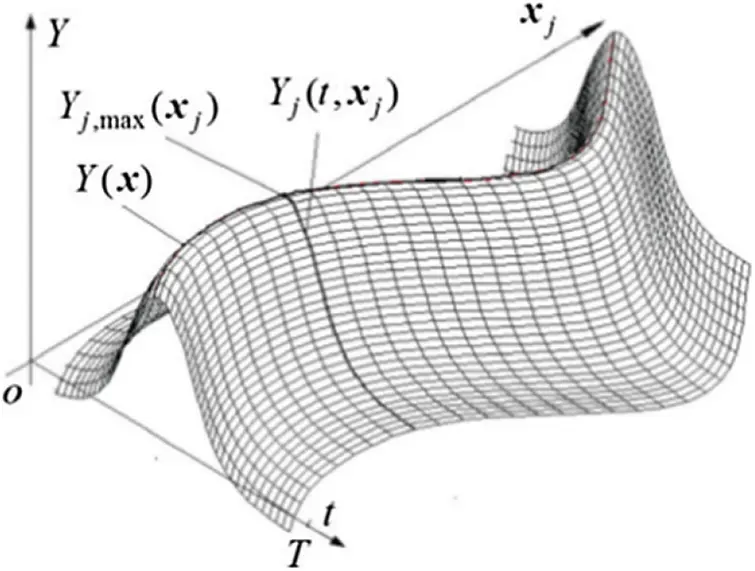Figure 1: Basic thought of DTR meta-modeling

As depicted in Fig. 1, in the time interval [0, T], the time-varying responses Yj(t, xj) are generated for input samples xj with a determined distribution. In view of the dynamic extremum thought, the extremum response Yj,max(xj) is extracted to form the extremum sample set as Eq. (1).

{(xj,Yj,max(xj))|Yj,max(xj)=Maxt[Yj(t,xj)],t[0,T],j=1,2,,l}(1)

With implicit mapping function ϕ(⋅) to map each input sample to high-dimensional feature space, the extremum sample set is fitted by a DTR meta-model function f(x, ω) to approximate the nonlinear relationship between input variables xn and extremum response Y(x)∈ , as shown in Eq. (2).

Y(x)=f(x,ω)={xjTRnYj,max(xj)}=ωϕ(x)+b(2)

where ω = [ω1, ω2, …, ωL] is the weight coefficient vector, ϕ(x) = [ϕ1(x), ϕ2(x), …, ϕL(x)]T represents the feature space and b the bias coefficient. Therein, L denotes the dimension degree of feature space.

Obviously, the feasibility of DTR meta-model is dependent on the weight coefficient vector ω and the bias coefficient b. Thus, the problem of DTR meta-modeling is converted to training for the optimal weight coefficient vector ω and bias coefficient b by minimizing the structural risk. The empirical risk and confidence risk are combined to constitute the structural risk as Eq. (3).

Rstr=Remp+CRcon(3)

where the empirical risk Remp =j=1lE(Yj,max(xj),f(xj,ω)), in which E(Yj,max(xj), f(xj, ω)) represents the error function; the confidence risk Rcon =|ω|2, in which |ω|2 represents the complexity of DTR meta-model; C is the penalty coefficient to achieve the trade-off between complexity and losses.

To enhance the generalization ability of DTR meta-model, the quadratic tube error function is introduced as Eq. (4).

Et2(Yj,max(xj),f(xj,ω))=(Et(Yj,max(xj),f(xj,ω)))2(4)

in which linear tube error function is termed as Eq. (5).

Et(Yj,max(xj),f(xj,ω))={0,|Yj,max(xj)f(xj,ω)|<ε|Yj,max(xj)f(xj,ω)|ε,|Yj,max(xj)f(xj,ω)|ε(5)

where ε signifies the loss insensitive degree, representing the error threshold of sample data.

Therefore, with the slack variable ξj introduced to represent the losses, the structural risk minimization problem is expressed as Eq. (6).

minj=1lξj2+C||ω||2s.t.  |Yj,max(xj)f(xj,ω)|ε+ξj,ξj0,j=1,2,,l(6)

In the optimization process, the kernel function ψ(xi, xj) satisfying Mercer's theorem is introduced for scalar product in the feature space. Then the dual problem is derived as Eq. (7).

maxj=1lYj,max(xj)γjεj=1l|γj|12i,j=1lγiγj(ψ(xi,xj)+1Cδij)s.t.  j=1lγj=0  j=1,2,,l(7)

By solving the dual problem, the DTR meta-model function is retrieved with the optimal solution as Eq. (8).

{Y(x)=f(x)=xjSVγjψ(xj,x)+bx=[x1,x2,,xl],xj=[xj1,xj2,,xjr]T(8)

where γj* and b* indicate the optimal solution; r the amount of input variables; l the amount of samples; SV the support vector for a given sample set.

2.1.2 Dynamic WTR, DWTR

In DTR meta-modeling, the nonlinear fitting ability relies on the kernel function, as the scalar product in implicit feature space is directly expressed by the kernel trick. However, the Gaussian radial basis function (RBF) kernel function, as the conventional kernel function for TR meta-modeling, is insufficient when applied to approximate the extremum response in stochastic flutter assessment owing to high nonlinearity and strong interaction between fluid domain and structure domain. To improve the nonlinear fitting ability of DTR meta-model, a dynamic wavelet TR meta-model is further developed by employing the wavelet basis function as kernel function. The DWTR meta-modeling is introduced as follows.

In light of the wavelet analysis theory , a set of complete bases in L2 space are first obtained by the dilation and translation of wavelet basis functions, and then the extremum response is approximated subsequently. Therein, the prominent merit of wavelet in time-frequency localization can effectively improve the approximation accuracy of DWTR. Based on the theorem of translation-invariant kernel , the wavelet kernel function can be expressed as Eq. (9).

ψ(xi,xj)=k=1rh(xikxjkσ)(9)

where h(⋅) is the mother wavelet function; xik, xjk the kth input variable of ith and jth sample, respectively; σ the dilation factor.

Considering the rapid attenuation characteristics, the continuous Morlet wavelet function in Eq. (10) is substituted as the mother wavelet function.

h(x)=cos(ω0x)exp(x22)(10)

where ω0 denotes the frequency coefficient. Then the Morlet wavelet kernel function is derived as Eq. (11).

ψ(xi,xj)=k=1rcos(ω0xikxjkσ)exp(||xixj||22σ2)(11)

With the Morlet wavelet kernel function to replace the Gaussian RBF kernel function, the DWTR meta-model is structured as Eq. (12).

{Y(x)=f(x)=xjSVγjk=1rcos(ω0xjkxkσ)exp(||xjx||22σ2)+bx=[x1,x2,,xl],xj=[xj1,xj2,,xjr]T(12)

where xk denotes the kth input variable.

2.2 BA-DWTR

The performance of aforementioned DWTR meta-model is largely reliant on three undetermined parameters (C, σ, ε): (1) The penalty coefficient C balances the empirical risk and confidence risk; (2) The dilation factor σ determines the wavelet fidelity; (3) The loss insensitive degree ε controls the error threshold of sample data. To ensure the prediction performance of DWTR, an improved bat algorithm (BA) is developed to search for the optimal parameters of meta-model, called as BA-DWTR.

BA is a metaheuristic searching method combining the standard particle swarm optimization and harmony search, which possesses fast-convergence and high-precision virtues . Nevertheless, the regular BA confronts the problems of premature convergence and slow convergence, owing to the inflexible flight behavior in global exploitation and the time-invariant perturbation in local exploration. To address this issue, the adaptive flight mechanism and shrinking Gaussian perturbation are designed for BA algorithm, which enhances the global search capability and elevates the convergence rate. The basic procedures of BA-DWTR modeling are summarized as follows.

2.2.1 Global Exploitation by Adaptive Flight Mechanism

In global exploitation, the bat position X is composed of the loss insensitive degree, the penalty coefficient and the dilation factor, and each bat position Xi is a potential solution for optimal parameters in BA-DWTR meta-model. By adopting the training error of BA-DWTR as the fitness value, all bats prey for the optimum in search space with the ruled collaborative flight. Considering the flight speed is relevant to the distance between the bat position and the best solution, the adaptive flight mechanism is proposed to adjust the bat flight behavior, then the update rules of bat velocities and positions can be given by Eq. (13).

{ Vit+1=witVit+(XitXt)fiXit+1=Xit+Vit+1s.t.{ fi=fmin+(fmaxfmin)βwit=w0(1exp(λ||XitXt||))(13)

where i denotes the ith bat; t the current time step; Vi the ith bat velocity; Xi the ith bat position; X* the current best solution; fi in a range [fmin, fmax] is the pulse frequency to control the velocity increment; β the random vector drawn from the uniform distribution U(0, 1); wi the adaptive weight that reflects the degree of the current velocity inheriting the previous velocity; w0 1 the initial adaptive weight; λ>0 the distance coefficient depending on the scale of solution space; ||XiX*|| the distance between the current bat position and the current best solution in search space.

2.2.2 Local Exploration by Shrinking Gaussian Perturbation

In local exploration, once a candidate solution is selected among the current best solutions, the bat position is updated through random perturbation to the current best objective. With consideration of the time-variant characteristic and distribution of the current best objective, the shrinking factor-based Gaussian random walk is taken as the perturbation approach. The perturbation formula of the current best objective is shown as Eq. (14).

Xtempt+1=Xtempt+ηtξGAts.t.ηt=η0(1tTmax)θ(14)

where t denotes the current time step; Tmax the maximum time step; Xtemp the temporary best solution; ξG the random number obeying Gaussian distribution N(0, 1); A the current average loudness; η the shrinking factor changing with iteration number to restrict the perturbation degree; 0<η0 < 1 the initial shrinking factor; θ>1 the positive integer.

During the global exploitation and local exploration, the loudness and pulse emission rate of bat algorithm would be updated when a bat approaches to the best solution, the corresponding update formula is presented as Eq. (15).

{Ait+1=αAitrit+1=ri0(1exp(γt))(15)

where i represents the ith bat; t the current time step; Ai the loudness of current bat; ri the pulse emission rate of the current bat; ri0 the limit pulse emission rate of the current bat; 0<α<1, β>0 are two constants.

On account of the flexibility of adaptive flight mechanism and time-variant random characteristic of shrinking Gaussian perturbation, the best meta-model parameters (C*, σ*, ε*) can be determined effectively by the improved BA, and then the BA-DWTR meta-model is established accordingly with high approximation accuracy. Hence, the BA-DWTR is assured to enhance the computing accuracy and efficiency for stochastic flutter assessment of turbomachinery.

2.3 Stochastic Flutter Assessment Procedure of BA-DWTR

To enhance the computing accuracy and efficiency of stochastic flutter assessment, the BA-DWTR is developed by incorporating the simplified calculation ability of dynamic extremum thought, local precise description ability of wavelet TR and global searching ability of the improved BA. Based on the proposed BA-DWTR, the stochastic flutter assessment procedure is shown in Fig. 2.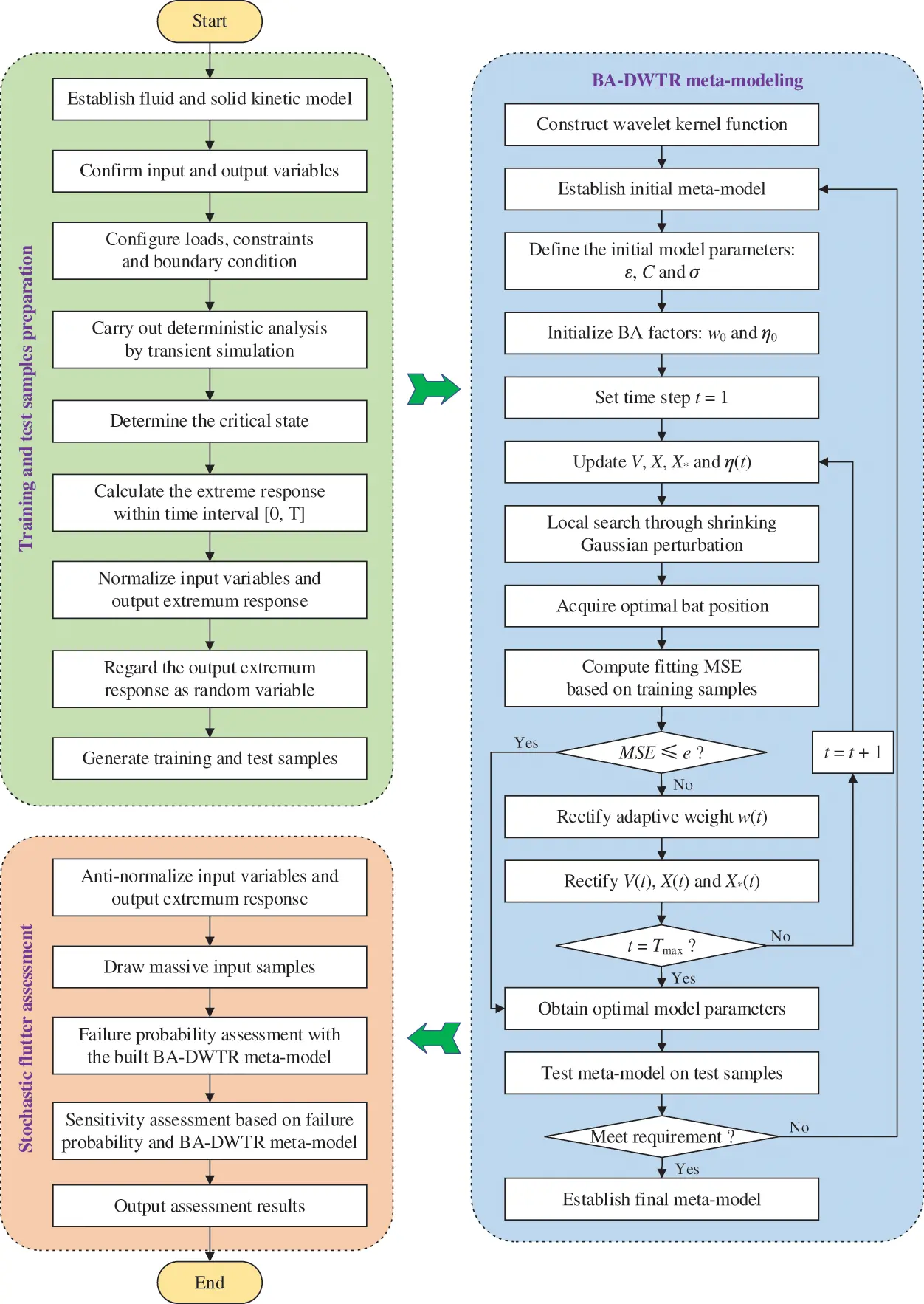Figure 2: Framework of stochastic flutter assessment

As illustrated in Fig. 2, the stochastic flutter assessment procedure with BA-DWTR, comprising training & testing samples preparation, BA-DWTR meta-modeling and stochastic flutter assessment, is introduced as follows:

(1)   Establish deterministic flutter model and configure input random variables (boundary conditions, dynamic loads, constraint conditions, material properties, model variations, etc.).

(2)   Acquire dynamic output responses by imposing input variables into deterministic flutter simulation.

(3)   Extract the extremum value of dynamic output responses to construct training & testing samples.

(4)   Perform BA-DWTR meta-modeling with the generated samples and meta-model function.

(5)   Accomplish the stochastic flutter assessment by the built BA-DWTR meta-model.

In Step (5), the stochastic flutter assessment mainly includes stochastic flutter failure probability assessment and sensitivity assessment, which is to quantify the flutter failure risk and to grade the influence degree of input random variables on flutter failure risk. With massive sampling based on the established BA-DWTR meta-model, the stochastic flutter assessment is implemented by the following expressions [64,65] in Eq. (16).

{ Pf=1Nj=1Nλf[g(xj)]=NfNSk=Pfμk=E(λf[g(x)]xkμkσk2)s.t.λf[g(x)]={ 1,g(x)00,g(x)>0(16)

where the limit state function g(x)=Y(x)−Ymin, in which Y(x) is the extremum response approximated by BA-DWTR meta-model and Ymin is the allowable minimum of extremum response; λf[g(x)] is the failure logic function, in which g(x) ≤ 0 indicates the failure domain and vice versa; Pf the stochastic flutter failure probability; Nf , N the amount of failure and total samples, respectively; Sk the sensitivity degree of kth input random variable; E(⋅) the mean value function; μk, σk2 the mean and variance of kth input random variable, respectively .

3  Stochastic Flutter Assessment of Aeroengine Compressor Blade

In this section, regarding the time-varying loads and boundary conditions, the stochastic flutter assessment of a typical aeroengine compressor blade [67,68] is conducted to verify the proposed BA-DWTR meta-modeling approach. The computing environment is an Intel(R) Core(TM) Desktop Computer (3.20 GHz CPU and 16 GB RAM).

3.1 Material Preparations

As a typical turbomachinery, the aeroengine compressor is subjected to multiple time-varying loads and boundary conditions, which is prone to cause significant change on stochastic flutter behavior . Therefore, the blade of a typical aeroengine compressor is taken as the object to investigate the stochastic flutter assessment of turbomachinery. By adopting 20-node hexahedron element technique [72,73], the blade finite element (FE) model with 800 elements and 4725 nodes is established as shown in Fig. 3. The titanium alloy is selected as the blade material, whose material density, elastic modulus and Poisson ratio are set as 4.44 g/cm3, 112 GPa and 0.3, respectively [74,75]. To obtain the blade vibrating features, the solid kinetic analysis is performed at 100% rotative rate, the vibrating frequency is gained and the first modal shape is depicted in Fig. 4. Similarly, the vibrating frequencies at different rotative rates are acquired as shown in Fig. 5, which will be considered as the time-varying loads spectrum in the following stochastic flutter assessment.Figure 3: Finite element modelFigure 4: First modal shape of the blade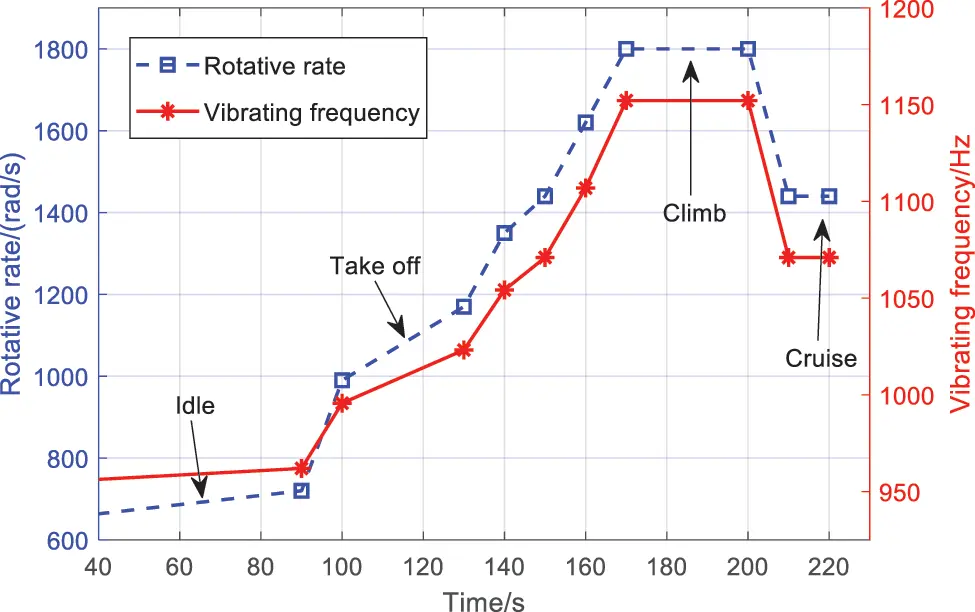In one trial of deterministic flutter simulation, the rotative rate, vibrating frequency, total temperature at rotor inlet, total pressure at rotor inlet and static pressure at rotor outlet determine the output response, but these parameters have great fluctuation in operating condition, which results in the fluctuation of the output response. To measure the stochastic uncertainties of blade operating environment, the boundary conditions (i.e., total temperature at rotor inlet T*in, total pressure at rotor inlet p*in and static pressure at rotor outlet pout) and time-varying loads (i.e., rotative rate n and vibrating frequency f) are reckoned as input random variables. The distribution traits of boundary conditions and time-varying loads are listed in Table 1. Note that all selected variables are reciprocally independent and obey Gaussian distribution.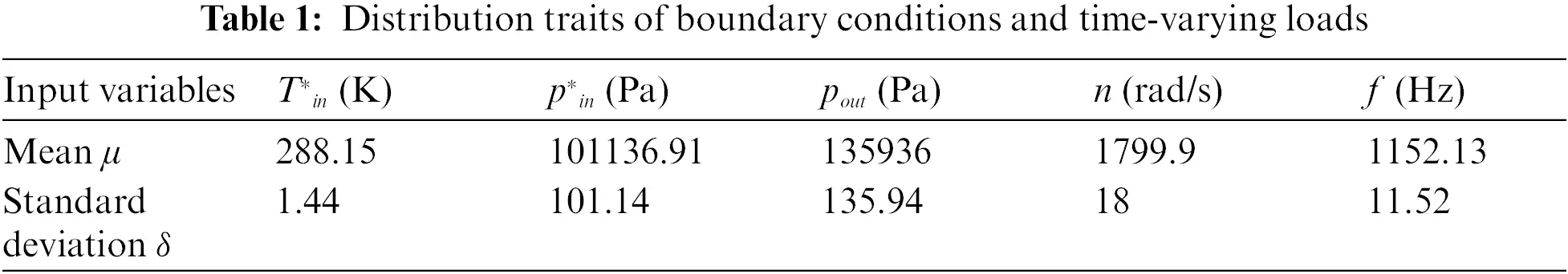3.2 Deterministic Flutter Simulation

To acquire the time-varying flutter response, the deterministic analysis was conducted by steady flow simulation and unsteady flow simulation. Through the steady flow simulation, the steady flow field at each working condition was determined and transferred to the unsteady flow simulation. Then the unsteady flow simulation was performed by taking the steady flow field as the initial flow field. The aerodynamic modal damping ratio (AMDR) corresponding to the input variables was obtained as the output response to construct the input-output samples for subsequent BA-DWTR meta-modeling.

On account of the cyclic symmetry of turbomachinery and the periodicity of blade vibration, the two blade flow passages are modeled for flow simulation. Considering the body-fitted hexahedron grid technique, the blade flow passage is discretized into 136,416 elements and 150,460 nodes, as shown in Fig. 6. By imposing the inlet total temperature and the inlet total pressure as 288.15 K and 101,325 Pa, the steady flow simulation is performed with given rotative rate (70%, 80%, 90% and 100% rotative rate), and the static pressure distribution at 100% rotative rate is displayed in Fig. 7. By calculating the area-averaged total pressure at the inlet surface and the outlet surface, the total pressure ratio was obtained. Correspondingly, the performance map of the blade was measured for each given rotational speed characteristic, as shown in Fig. 8.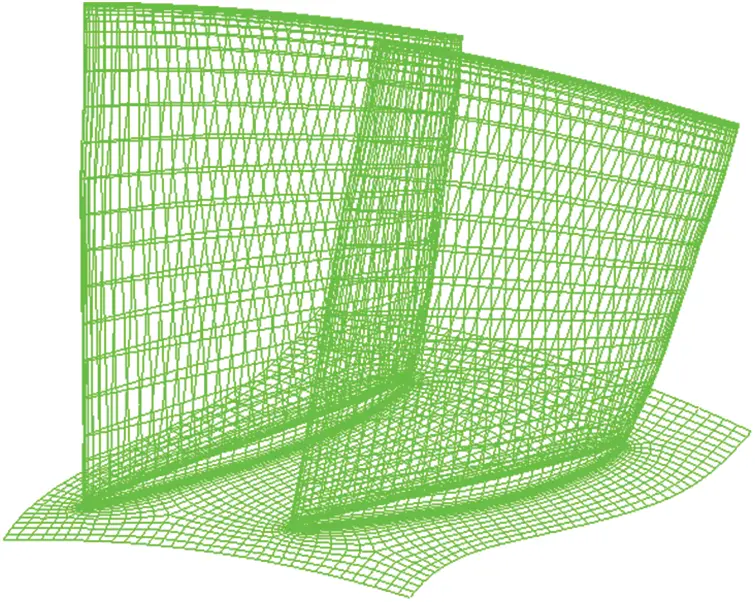Figure 6: Computational grid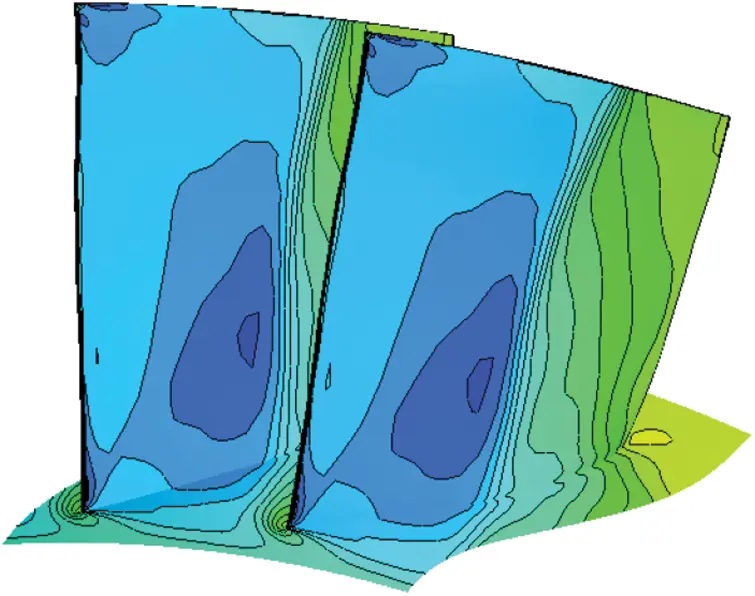Figure 7: Static pressure distribution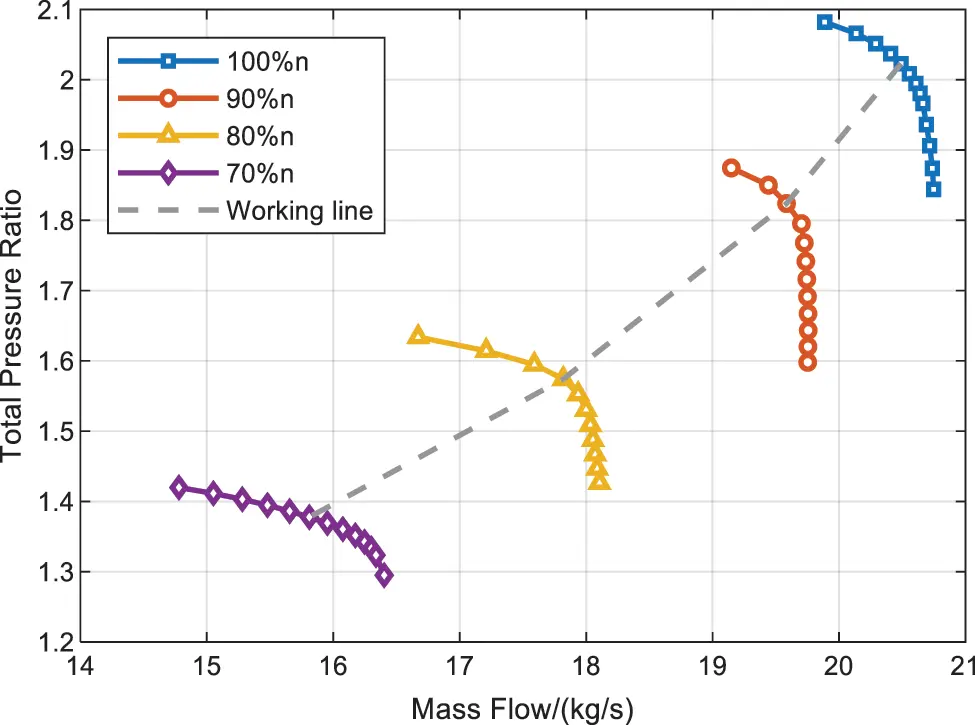Fig. 8 manifests that the total pressure ratio varies with the flow rate at a fixed rotative rate. When the flow rate decreases to a certain extent, the pressure ratio does not increase any more, the rotor enters an unstable stall working state. On the contrary, when the flow rate increases to a certain extent, the total pressure ratio would decrease rapidly, the rotor reaches the choke margin. The working line is near the stall margin, as shown in Fig. 8. Note that the stall margin is the connecting line of stall working points. Since the working line is within the stall margin, the working states beyond the stall margin are not considered. The detailed information of the operating conditions along the working line are as follows: The static pressure at rotor outlet are 95, 103, 112, 118, 130 and 136 kPa for 55%, 65%, 75%, 80%, 90% and 100% rotative rate, respectively; The mass flow at rotor outlet are 14.11, 15.31, 16.94, 17.85, 19.61 and 20.49 kg/s for 55%, 65%, 75%, 80%, 90% and 100% rotative rate, respectively.

Figure 8: Performance map of rotor 37

Considering time-varying loads spectrum and steady flow simulation, the unsteady flow simulation was executed using the energy method in the most unstable vibration mode of 1 nodal diameter , where the inter-blade phase angle (IBPA) is 10 deg. Herein, the flow analysis is governed by three-dimensional Reynolds averaged Navier-Stokes (RANS) equations with k-ε turbulent model, and is performed by dynamic grid technique and double channel harmonic method based on phase correction. The detailed turbulence parameters are Cμ = 0.09, Cε1 = 1.44, Cε2 = 1.92, σk = 1.0, σε = 1.3. In light of energy method, the AMDR is defined as the ratio of the aerodynamic work in an oscillating cycle to the maximal vibrating kinetic energy and measured by the integral of the aerodynamic work on blade surface nondimensionalized by the blade vibrating frequency and the modal amplitude. By expressing AMDR under each operating condition on the compressor rotor characteristic map, the performance map of rotor blade is illustrated in Fig. 9. At about t =170 s, the AMDR reaches the minimum, whose aerodynamic work distribution is shown in Fig. 10. Moreover, along the working line marked in Fig. 8, assuming a smooth transition process between each operating condition, the AMDR is acquired as shown in Fig. 11, which illustrates the AMDR greater than zero with the given time-varying loads. Note that the flutter failure will be induced when the AMDR is less than zero, which means the allowable minimum of extremum response Ymin =0.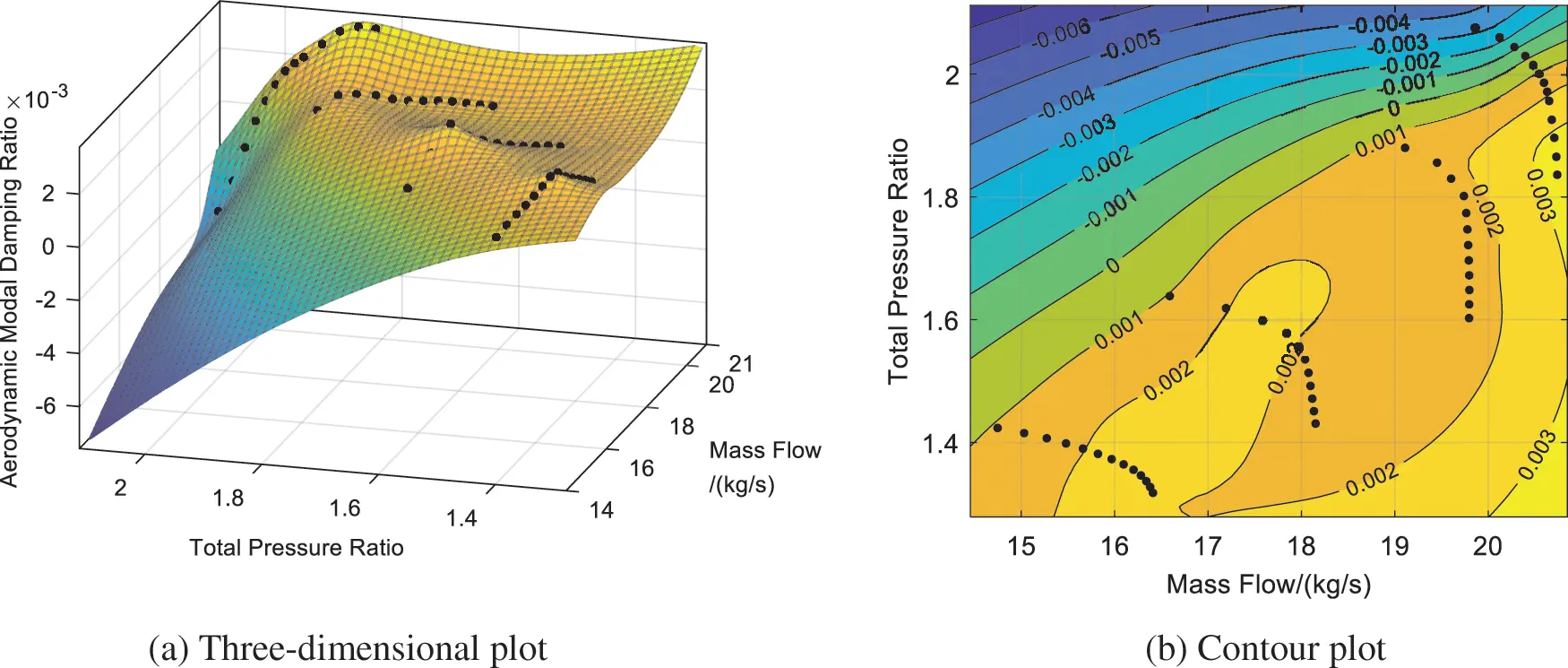Figure 9: Performance map of rotor blade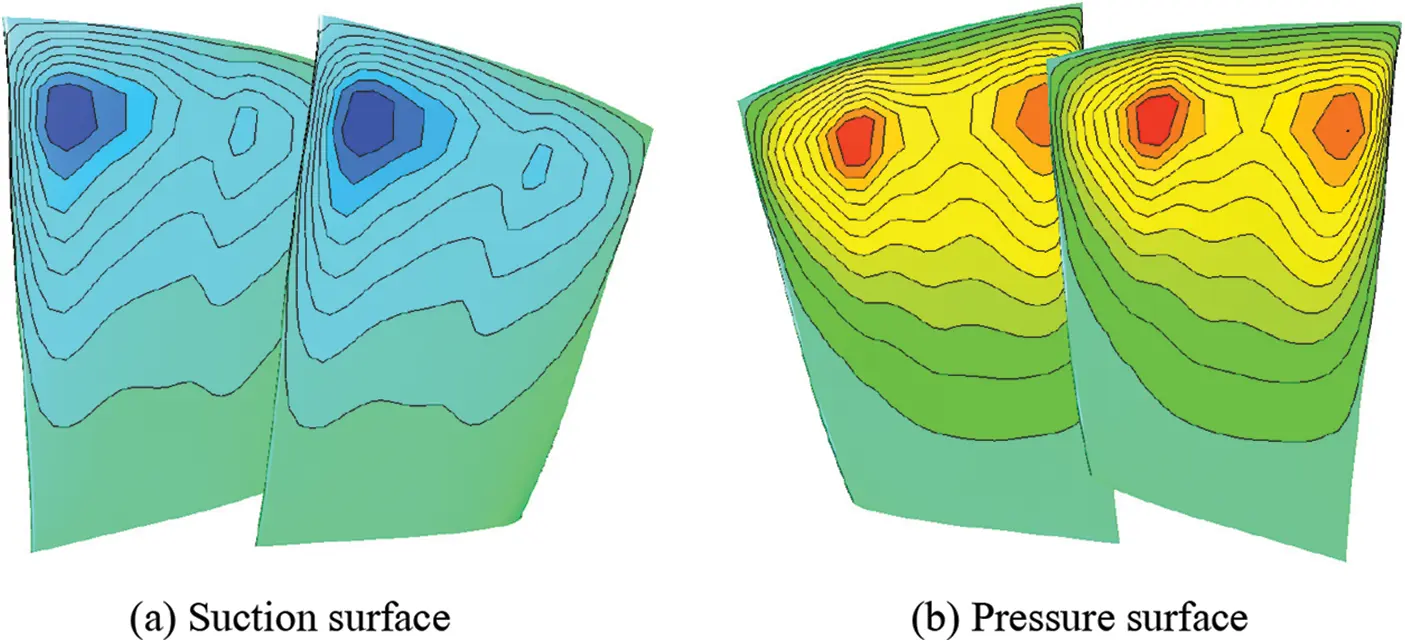Figure 10: Aerodynamic work on blade surface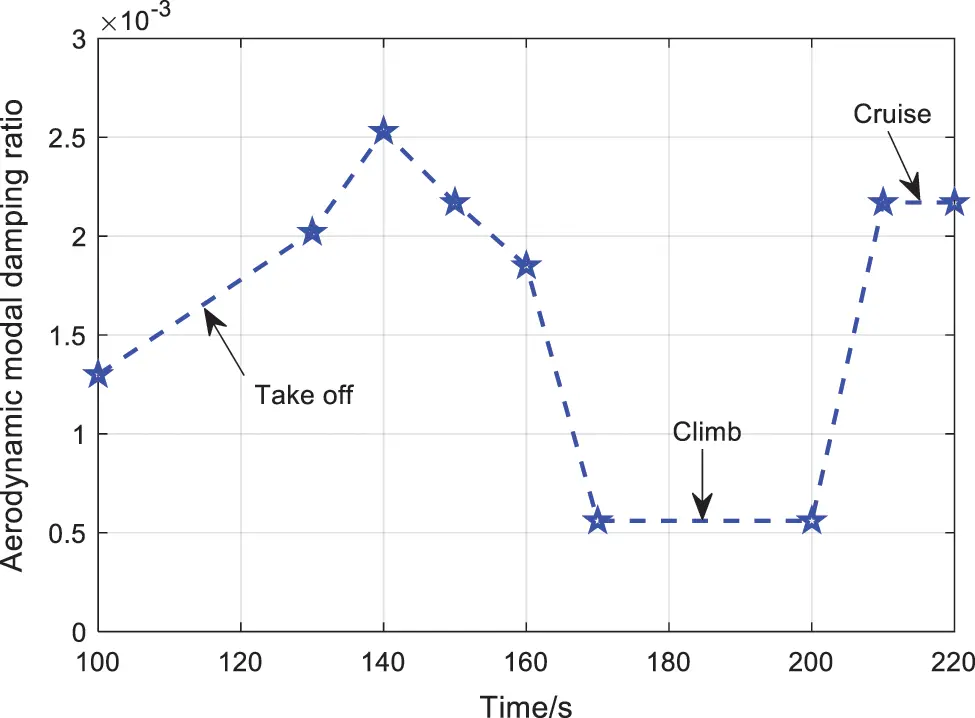Figure 11: Time-varying response of aerodynamic modal damping ratio

3.3 BA-DWTR Meta-Modeling

Based on the distribution traits of boundary conditions and time-varying loads in Table 1, 140 groups of input samples (40 training samples and 100 testing samples) are extracted by Latin hypercube sampling technique. Importing these samples into the deterministic flutter simulation, the output response (AMDR) corresponding to the input variables are obtained to train the BA-DWTR meta-model and test the estimation precision of the built BA-DWTR meta-model. With the trained BA-DWTR to express the nonlinear relationship between input variables and output response, the response nephographs of partial input variables are depicted in Fig. 12. The estimation performance of BA-DWTR is verified by testing samples and compared with other meta-models (RS, DTR and DWTR), as shown in Fig. 13 and Table 2.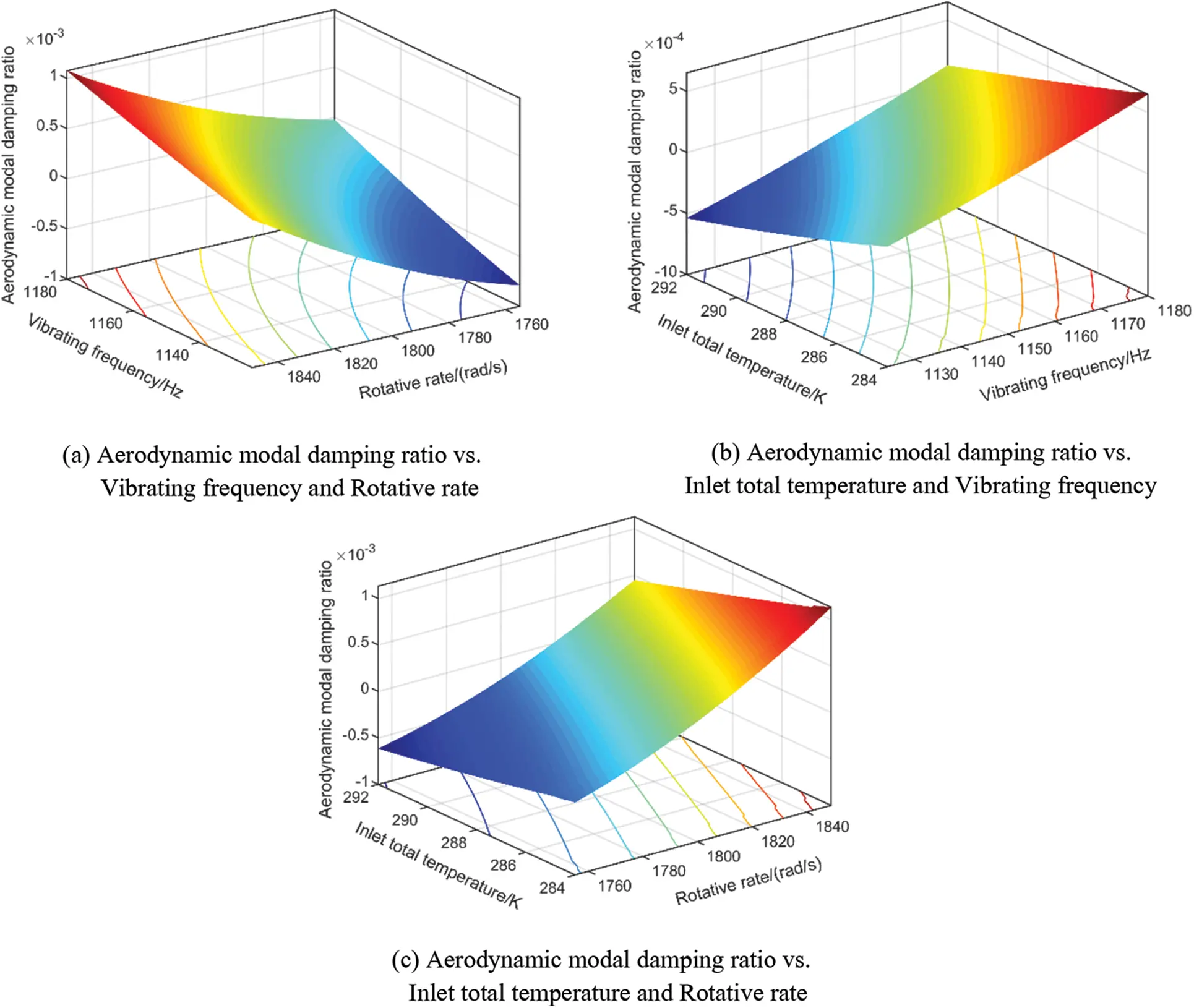Figure 12: Response nephographs of partial input variables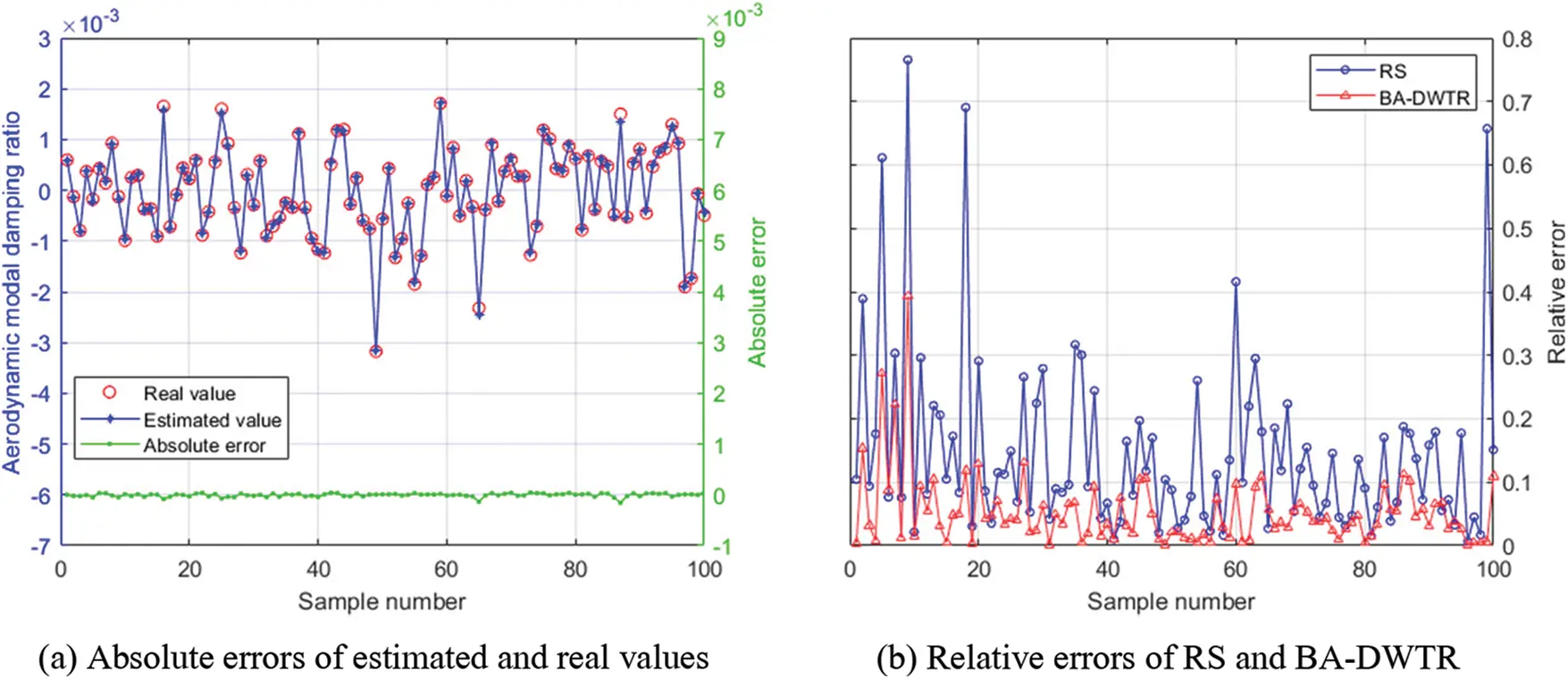Figure 13: Prediction results of BA-DWTR model
Note: That the absolute error and relative error of each sample are calculated by ŷj − yj and |(ŷj − yj)/yj|, respectively, where ŷj and yj are the estimated value and real value of output response.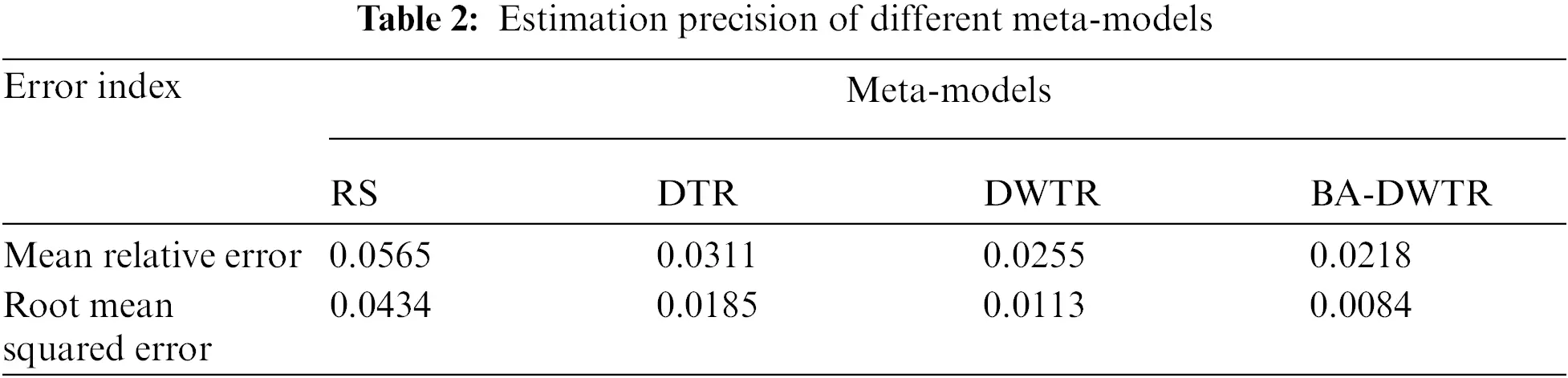3.4 Stochastic Flutter Assessment

By Latin hypercube sampling based on the distribution traits of boundary conditions and time-varying loads, the BA-DWTR meta-model replacing the deterministic flutter model was simulated 10000 times by MC simulation. The output responses are gained and the distribution features of output response are depicted in Fig. 14. As illustrated in Fig. 14, the AMDR nearly follows a Gaussian distribution with mean value of 0.0006527 and standard deviation of 0.0002858.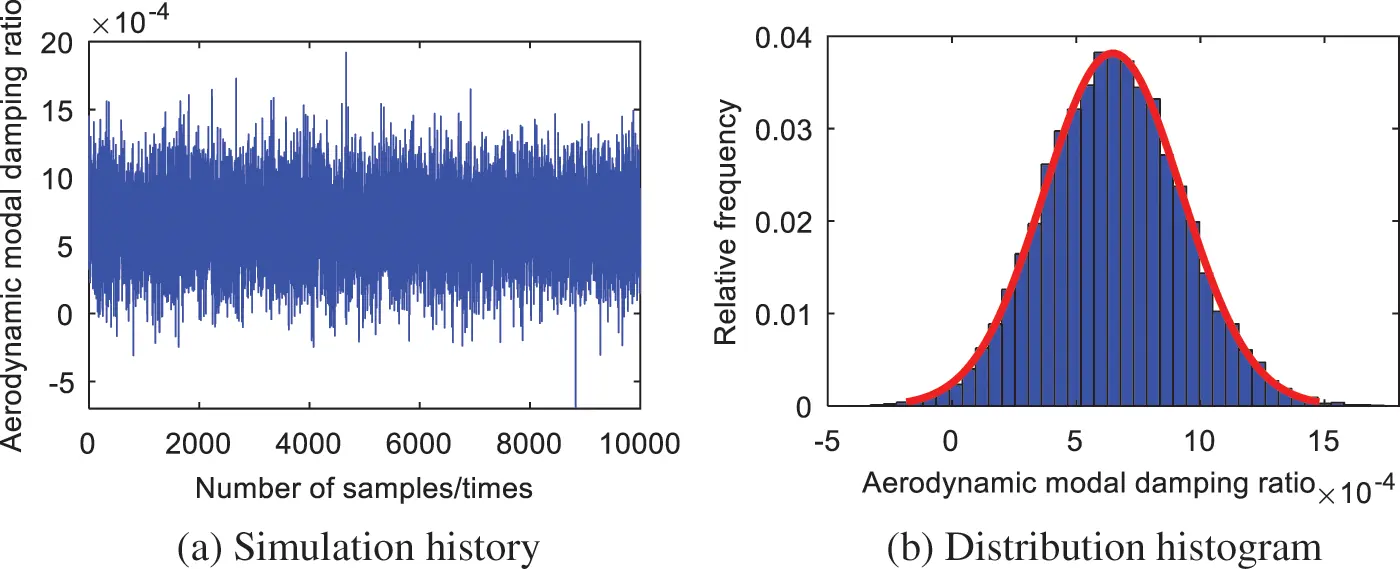Figure 14: Distribution features of output response

In light of the stochastic flutter assessment model in Eq. (16) and the 10000 times simulation results, the stochastic flutter failure probability assessment and sensitivity assessment are fulfilled. The stochastic flutter failure probability is calculated as 0.95%, and the sensitivities and influence probabilities of input variables on output response are revealed in Fig. 15. As shown in Fig. 15, the total temperature at rotor inlet, vibrating frequency and rotative rate pose the main effects on failure probability with influence probabilities of 64%, 19% and 14%, respectively. In addition, the failure probability is positively correlated with the total temperature at rotor inlet and the static pressure at rotor outlet, but negatively correlated with other variables.Figure 15: Sensitivities and influence probabilities of input variables.

3.5 Methods Validation

For the validation of the proposed BA-DWTR, the stochastic flutter assessment of aeroengine compressor blade is also investigated by direct MC simulation, RS, DTR and DWTR. Considering the rationale of methods comparison, all methods are assigned equal computing tasks and allocated the same computing resources. In view of the same input variables as shown in Table 1, the stochastic flutter failure probability is retrieved through stochastic flutter assessment. The computational performance of stochastic flutter assessment is listed in Tables 3 and 4.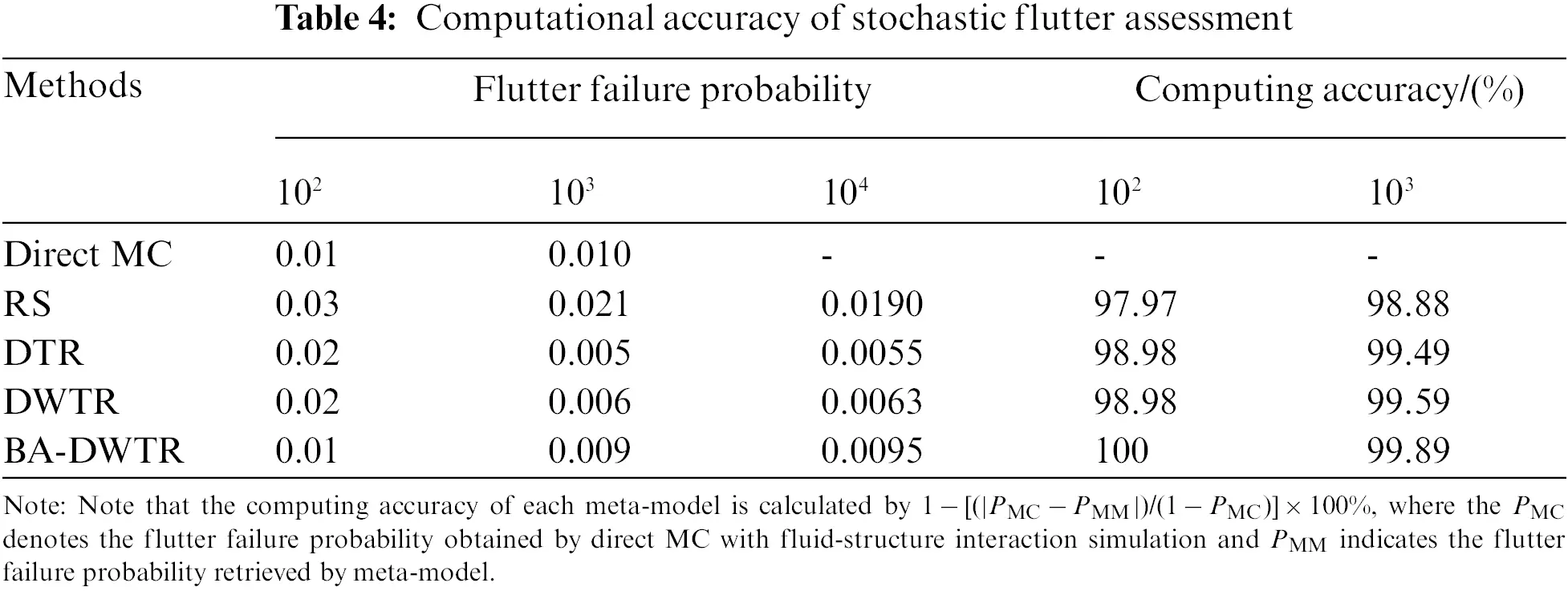As revealed in Table 3, the required sample amount and training time of BA-DWTR are less than RS, DTR and DWTR, and the four meta-models consumes far less simulation time for stochastic assessment than direct MC simulation. In the sight of both the training time and simulation time, the computing efficiency of the presented BA-DWTR is the highest, owing to: (1) the use of dynamic extremum thought makes BA-DWTR only extract the extremum response instead of full-scale time-varying responses in the time interval; (2) the local precise description ability of wavelet kernel function facilitates BA-DWTR to fit the training samples and cut down the required amount of training samples; (3) the improved BA accelerates the process to search the optimal parameters of BA-DWTR with adaptive flight strategy and shrinking Gaussian perturbation to avoid the blind search. Therefore, the BA-DWTR is demonstrated to hold the high computing efficiency in stochastic flutter assessment of turbomachinery.

As illustrated in Table 4, the computing accuracy of BA-DWTR is higher than RS, DTR and DWTR, and almost consistent with direct MC simulation, resulting from: (1) the BA-DWTR is trained based on TR with great nonlinear fitting ability and strong generalization ability; (2) the wavelet transformation technique-based wavelet kernel function boosts the nonlinear fitting ability of BA-DWTR; (3) the global searching ability of the improved BA avoids poor parameters of BA-DWTR to ensure the estimation precision. Hence, the BA-DWTR is proven to possess the high computing accuracy in stochastic flutter assessment of turbomachinery.

In summary, the comparison results validate that the proposed BA-DWTR can greatly enhance the computing efficiency with assured computing accuracy, and provide a feasible and effective way for the stochastic flutter assessment of turbomachinery.

4  Conclusions

In this paper, a dynamic meta-modeling approach (BA-DWTR) is developed to reveal the stochastic flutter behavior of turbomachinery. The dynamic extremum thought and the wavelet transform technique are employed to legitimately tackle with the large-dynamicity and high-nonlinearity issues induced by uncertain factors. To coordinate strong generalization ability and great nonlinear mapping ability, the bat algorithm is designed to search the optimal model parameters. The effectiveness of the presented approach has been validated by the stochastic flutter assessment of a typical aero-engine compressor blade. The distribution features and sensitivity factors of the aerodynamic modal damping ratio were achieved. Results show that there is 0.95% probability to trigger the flutter failure when the compressor reaches 100% rotative rate at about t = 170 s. The total temperature at rotor inlet and dynamic working loads (vibrating frequency and rotative rate) are the most critical design variables on the flutter failure probability since their effect probabilities of 64%, 19% and 14%, respectively. The negative control of total temperature at rotor inlet and the positive control of vibrating frequency and rotative rate can reduce the flutter failure probability. Through the comparison of methods (direct Monte Carlo simulation, response surface method, dynamic tube regression), the proposed BA-DWTR is demonstrated to possess high-accuracy and high-efficiency in stochastic flutter assessment.

Funding Statement: This paper is co-supported by the National Natural Science Foundation of China (Grants 51975028 and 52105136), China Postdoctoral Science Foundation (Grant 2021M690290) and the National Science and Technology Major Project (Grant J2019-IV-0016-0084). The authors would like to thank them.

Conflicts of Interest: The authors declare that they have no conflicts of interest to report regarding the present study.

## References

1. Campobasso, M. S., & Giles, M. B. (2003). Effects of flow instabilities on the linear analysis of turbomachinery aeroelasticity. Journal of Propulsion and Power, 19(2), 250-259. [Google Scholar] [CrossRef]
2. Forhad, M. M., Vishwakarma, P., & Xu, Y. (2011). Mu analysis for turbomachinery stall flutter. Turbo Expo: Power for land, sea, and air, GT2011–46624, 1439-1448. [Google Scholar] [CrossRef]
3. Li, X. Q., Song, L. K., & Bai, G. C. (2022). Recent advances in reliability analysis of aeroengine rotor system: A review. International Journal of Structural Integrity, 13(1), 1-29. [Google Scholar] [CrossRef]
4. Waite, J. J., & Kielb, R. E. (2016). The impact of blade loading and unsteady pressure bifurcations on low-pressure turbine flutter boundaries. Journal of Turbomachinery, 138(4), 41002. [Google Scholar] [CrossRef]
5. Meng, D., Yang, S., Zhang, Y., & Zhu, S. P. (2019). Structural reliability analysis and uncertainties-based collaborative design and optimization of turbine blades using surrogate model. Fatigue & Fracture of Engineering Materials & Structures, 42(6), 1219-1227. [Google Scholar] [CrossRef]
6. Zhang, Y. F., Liu, T., & Zhang, W. (2020). Nonlinear resonant responses, mode interactions, and multitime periodic and chaotic oscillations of a cantilevered pipe conveying pulsating fluid under external harmonic force. Complexity, 2020, 9840860. [Google Scholar] [CrossRef]
7. Liu, T., Zhang, W., Mao, J. J., & Zheng, Y. (2019). Nonlinear breathing vibrations of eccentric rotating composite laminated circular cylindrical shell subjected to temperature, rotating speed and external excitations. Mechanical Systems and Signal Processing, 127, 463-498. [Google Scholar] [CrossRef]
8. Zhang, W., Zheng, Y., Liu, T., & Guo, X. Y. (2019). Multi-pulse jumping double-parameter chaotic dynamics of eccentric rotating ring truss antenna under combined parametric and external excitations. Nonlinear Dynamics, 98(1), 761-800. [Google Scholar] [CrossRef]
9. Zhao, T. Y., Ma, Y., Zhang, H. Y., Pan, H. G., & Cai, Y. (2021). Free vibration analysis of a rotating graphene nanoplatelet reinforced pre-twist blade-disk assembly with a setting angle. Applied Mathematical Modelling, 93(10), 578-596. [Google Scholar] [CrossRef]
10. Li, Z., Jiang, J., & Tian, Z. (2017). Stochastic dynamics of a nonlinear misaligned rotor system subject to random fluid-induced forces. Journal of Computational and Nonlinear Dynamics, 12(1), 011004. [Google Scholar] [CrossRef]
11. Kumar, P., & Narayanan, S. (2009). Nonlinear stochastic dynamics, chaos, and reliability analysis for a single degree of freedom model of a rotor blade. Journal of Engineering for Gas Turbines and Power, 131(1), 012506. [Google Scholar] [CrossRef]
12. Beran, P., Stanford, B., & Schrock, C. (2017). Uncertainty quantification in aeroelasticity. Annual Review of Fluid Mechanics, 49, 361-386. [Google Scholar] [CrossRef]
13. Desai, A., & Sarkar, S. (2010). Uncertainty quantification and bifurcation behavior of an aeroelastic system. Fluids Engineering Division Summer Meeting, FEDSM-ICNMM2010-30050, 1177-1187. [Google Scholar] [CrossRef]
14. Pourazarm, P., Caracoglia, L., Lackner, M., & Modarres-Sadeghi, Y. (2016). Perturbation methods for the reliability analysis of wind-turbine blade failure due to flutter. Journal of Wind Engineering and Industrial Aerodynamics, 156, 159-171. [Google Scholar] [CrossRef]
15. Meng, D., Wang, H., Yang, S., Lv, Z., & Hu, Z. (2022). Fault analysis of wind power rolling bearing based on EMD feature extraction. Computer Modeling in Engineering & Sciences, 130(1), 543-558. [Google Scholar] [CrossRef]
16. Kielb, R. E., Hall, K. C., Hong, E., & Pai, S. S. (2006). Probabilistic flutter analysis of a mistuned bladed disks. ASME Turbo Expo 2006: Power for land, sea, and air, GT2006-90847, 1145-1150. [Google Scholar] [CrossRef]
17. Reddy, T. S. R., Mital, S., Stefko, G. (2004). Probabilistic aeroelastic analysis of turbomachinery components. 19th AIAA Applied Aerodynamics Conference, 1453. Anaheim, CA, USA.
18. Vinogradov, K. A., Kretinin, G. V., Leshenko, I. A., Otriakhina, K. V., Fedechkin, K. S. et al. (2018). Robust multiphysics optimization for fan blade aerodynamic efficiency, structural properties and flutter sensitivity. ASME Turbo Expo 2018: Turbomachinery Technical Conference and Exposition, Oslo, Norway. DOI 10.1115/GT2018-76816. [CrossRef]
19. Tateishi, A., Watanabe, T., Himeno, T., Aotsuka, M., Murooka, T. (2016). Statistical sensitivity study of frequency mistuning on the prediction of the flutter boundary in a transonic fan. ASME Turbo Expo 2016: Turbomachinery Technical Conference and Exposition, Seoul, South Korea. DOI 10.1115/GT2016-57295. [CrossRef]
20. Stapelfeldt, S., & Vahdati, M. (2018). On the importance of engine-representative models for fan flutter predictions. Journal of Turbomachinery, 140(8), 081005. [Google Scholar] [CrossRef]
21. Cao, D., & Bai, G. (2020). A study on aeroengine conceptual design considering multi-mission performance reliability. Applied Sciences, 10(13), 4668. [Google Scholar] [CrossRef]
22. Gao, J. X., & Yuan, Y. P. (2020). Probabilistic modeling of stiffness degradation for fiber reinforced polymer under fatigue loading. Engineering Failure Analysis, 116, 104733. [Google Scholar] [CrossRef]
23. Gao, J. X., Yuan, Y. P., & Xu, R. X. (2021). A framework for fatigue life prediction of materials under the multi-level cyclic loading. Engineering Failure Analysis, 127, 105496. [Google Scholar] [CrossRef]
24. Brahimi, F., & Ouibrahim, A. (2016). Blade dynamical response based on aeroelastic analysis of fluid structure interaction in turbomachinery. Energy, 115, 986-995. [Google Scholar] [CrossRef]
25. Zhang, W., Xu, Y., Su, D., & Gao, Y. (2019). Flutter analysis of tandem cascades based on a fluid-structure coupling method. Journal of Aerospace Engineering, 32(2), 04018147. [Google Scholar] [CrossRef]
26. Rendu, Q., Aubert, S., & Ferrand, P. (2020). Numerical identification of mechanisms triggering 2D choke flutter in transonic fan. Journal of Fluids and Structures, 97, 102879. [Google Scholar] [CrossRef]
27. Zhang, W., Gao, C., Liu, Y., Ye, Z., & Jiang, Y. (2015). The interaction between flutter and buffet in transonic flow. Nonlinear Dynamics, 82(4), 1851-1865. [Google Scholar] [CrossRef]
28. Kamakoti, R., & Shyy, W. (2004). Fluid-structure interaction for aeroelastic applications. Progress in Aerospace Sciences, 40(8), 535-558. [Google Scholar] [CrossRef]
29. Carstens, V., Kemme, R., & Schmitt, S. (2003). Coupled simulation of flow-structure interaction in turbomachinery. Aerospace Science and Technology, 7(4), 298-306. [Google Scholar] [CrossRef]
30. Meng, D., Li, Y., He, C., Guo, J., & Lv, Z. (2021). Multidisciplinary design for structural integrity using a collaborative optimization method based on adaptive surrogate modelling. Materials & Design, 206, 109789. [Google Scholar] [CrossRef]
31. Yang, F., & Ren, J. (2020). Reliability analysis based on optimization random forest model and MCMC. Computer Modeling in Engineering & Sciences, 125(2), 801-814. [Google Scholar] [CrossRef]
32. Narayanan, G. (2021). Probabilistic fatigue model for cast alloys of aero engine applications. International Journal of Structural Integrity, 12(3), 454-469. [Google Scholar] [CrossRef]
33. Huang, Y., Bai, G. C., Song, L. K., & Wang, B. W. (2020). Decomposed collaborative modeling approach for probabilistic fatigue life evaluation of turbine rotor. Materials, 13(14), 3239. [Google Scholar] [CrossRef]
34. Nahal, M., & Khelif, R. (2020). A finite element model for estimating time-dependent reliability of a corroded pipeline elbow. International Journal of Structural Integrity, 12(2), 306-321. [Google Scholar] [CrossRef]
35. Yang, Y. J., Wang, G., Zhong, Q., Zhang, H., & He, J. (2021). Reliability analysis of gas pipeline with corrosion defect based on finite element method. International Journal of Structural Integrity, 12(6), 854-863. [Google Scholar] [CrossRef]
36. Zhu, S. P., Liu, Q., Zhou, J., & Yu, Z. Y. (2018). Fatigue reliability assessment of turbine discs under multi-source uncertainties. Fatigue & Fracture of Engineering Materials & Structures, 41(6), 1291-1305. [Google Scholar] [CrossRef]
37. Zhu, S. P., Liu, Q., Peng, W., & Zhang, X. C. (2018). Computational-experimental approaches for fatigue reliability assessment of turbine bladed disks. International Journal of Mechanical Sciences, 142, 502-517. [Google Scholar] [CrossRef]
38. Liao, D., Zhu, S. P., Keshtegar, B., Qian, G., & Wang, Q. (2020). Probabilistic framework for fatigue life assessment of notched components under size effects. International Journal of Mechanical Sciences, 181, 105685. [Google Scholar] [CrossRef]
39. Li, X. Q., Song, L. K., & Bai, G. C. (2022). Vectorial surrogate modeling approach for multi-failure correlated probabilistic evaluation of turbine rotor. Engineering with Computers, 2022, 1-20. [Google Scholar] [CrossRef]
40. Zhi, P., Xu, Y., & Chen, B. (2019). Time-dependent reliability analysis of the motor hanger for EMU based on stochastic process. International Journal of Structural Integrity, 11(3), 453-469. [Google Scholar] [CrossRef]
41. Yue, H., Guo, C., Li, Q., Zhao, L., & Hao, G. (2020). Milling parameters optimization of Al-Li alloy thin-wall workpieces using response surface methodology and particle swarm optimization. Computer Modeling in Engineering & Sciences, 124(3), 937-952. [Google Scholar] [CrossRef]
42. Zhu, S. P., Keshtegar, B., Chakraborty, S., & Trung, N. T. (2020). Novel probabilistic model for searching most probable point in structural reliability analysis. Computer Methods in Applied Mechanics and Engineering, 366, 113027. [Google Scholar] [CrossRef]
43. Song, L. K., Bai, G. C., & Li, X. Q. (2021). A novel metamodeling approach for probabilistic LCF estimation of turbine disk. Engineering Failure Analysis, 120, 105074. [Google Scholar] [CrossRef]
44. Keshtegar, B., Heddam, S., Sebbar, A., Zhu, S. P., & Trung, N. T. (2019). SVR-RSM: A hybrid heuristic method for modeling monthly pan evaporation. Environmental Science and Pollution Research, 26(35), 35807-35826. [Google Scholar] [CrossRef]
45. Zheng, S., Jiang, A. N., & Feng, K. S. (2021). A reliability evaluation method for intermittent jointed rock slope based on evolutionary support vector machine. Computer Modeling in Engineering & Sciences, 129(1), 149-166. [Google Scholar] [CrossRef]
46. das Chagas Moura, M., Zio, E., Lins, I. D., & Droguett, E. (2011). Failure and reliability prediction by support vector machines regression of time series data. Reliability Engineering & System Safety, 96(11), 1527-1534. [Google Scholar] [CrossRef]
47. Song, L. K., Bai, G. C., Li, X. Q., & Wen, J. (2021). A unified fatigue reliability-based design optimization framework for aircraft turbine disk. International Journal of Fatigue, 152, 106422. [Google Scholar] [CrossRef]
48. Li, K., Kou, J., & Zhang, W. (2019). Deep neural network for unsteady aerodynamic and aeroelastic modeling across multiple mach numbers. Nonlinear Dynamics, 96(3), 2157-2177. [Google Scholar] [CrossRef]
49. Li, X. Q., Bai, G. C., Song, L. K., & Wen, J. (2021). Fatigue reliability estimation framework for turbine rotor using multi-agent collaborative modeling. Structures, 29, 1967-1978. [Google Scholar] [CrossRef]
50. Song, L. K., Wen, J., Fei, C. W., & Bai, G. C. (2018). Distributed collaborative probabilistic design of multi-failure structure with fluid-structure interaction using fuzzy neural network of regression. Mechanical Systems and Signal Processing, 104, 72-86. [Google Scholar] [CrossRef]
51. Teng, D., Feng, Y., Lu, C., Fei, C., & Liu, J. (2021). Novel kriging-based decomposed-coordinated approach for estimating the clearance reliability of assembled structures. Computer Modeling in Engineering & Sciences, 129(2), 1029-1049. [Google Scholar] [CrossRef]
52. Song, L. K., Bai, G. C., & Fei, C. W. (2019). Dynamic surrogate modeling approach for probabilistic creep-fatigue life evaluation of turbine disks. Aerospace Science and Technology, 95, 105439. [Google Scholar] [CrossRef]
53. Dutta, S. (2020). A sequential metamodel-based method for structural optimization under uncertainty. Structures, 26, 54-65. [Google Scholar] [CrossRef]
54. Bucher, C., & Most, T. (2008). A comparison of approximate response functions in structural reliability analysis. Probabilistic Engineering Mechanics, 23(2–3), 154-163. [Google Scholar] [CrossRef]
55. Giunta, A., Watson, L. (1998). A comparison of approximation modeling techniques-polynomial versus interpolating models. 7th AIAA/USAF/NASA/ISSMO Symposium on Multidisciplinary Analysis and Optimization, 4758. St. Louis, MO, USA.
56. Meng, D., Lv, Z., Yang, S., Wang, H., & Xie, T. (2021). A time-varying mechanical structure reliability analysis method based on performance degradation. Structures, 34, 3247-3256. [Google Scholar] [CrossRef]
57. Song, L. K., Fei, C. W., Bai, G. C., & Yu, L. C. (2017). Dynamic neural network method-based improved PSO and BR algorithms for transient probabilistic analysis of flexible mechanism. Advanced Engineering Informatics, 33, 144-153. [Google Scholar] [CrossRef]
58. Song, L. K., & Bai, G. C. (2020). Multi-surrogate collaboration approach for creep-fatigue reliability assessment of turbine rotor. IEEE Access, 8, 39861-39874. [Google Scholar] [CrossRef]
59. Meng, D., Xie, T., Wu, P., He, C., & Hu, Z. (2021). An uncertainty-based design optimization strategy with random and interval variables for multidisciplinary engineering systems. Structures, 32, 997-1004. [Google Scholar] [CrossRef]
60. Meng, D., Hu, Z., Guo, J., Lv, Z., & Xie, T. (2021). An uncertainty-based structural design and optimization method with interval taylor expansion. Structures, 33, 4492-4500. [Google Scholar] [CrossRef]
61. Abd Rahim, A. A., Abdullah, S., Singh, S. S. K., & Nuawi, M. Z. (2019). Reliability assessment on automobile suspension system using wavelet analysis. International Journal of Structural Integrity, 10(5), 602-611. [Google Scholar] [CrossRef]
62. Dai, H., & Cao, Z. (2017). A wavelet support vector machine-based neural network metamodel for structural reliability assessment. Computer-Aided Civil and Infrastructure Engineering, 32(4), 344-357. [Google Scholar] [CrossRef]
63. Yang, X. S. (2010). A new metaheuristic bat-inspired algorithm. Nature Inspired Cooperative Strategies for Optimization, pp. 65–74. Springer, Berlin, Heidelberg. DOI 10.1007/978-3-642-12538-6. [CrossRef]
64. Song, L. K., Fei, C. W., Wen, J., & Bai, G. C. (2017). Multi-objective reliability-based design optimization approach of complex structure with multi-failure modes. Aerospace Science and Technology, 64, 52-62. [Google Scholar] [CrossRef]
65. Song, L. K., Bai, G. C., & Fei, C. W. (2019). Probabilistic LCF life assessment for turbine discs with DC strategy-based wavelet neural network regression. International Journal of Fatigue, 119, 204-219. [Google Scholar] [CrossRef]
66. Song, L. K., Bai, G. C., & Fei, C. W. (2019). Multi-failure probabilistic design for turbine bladed disks using neural network regression with distributed collaborative strategy. Aerospace Science and Technology, 92, 464-477. [Google Scholar] [CrossRef]
67. Reid, L., Moore, R. D. (1978). Design and overall performance of four highly loaded, high speed inlet stages for an advanced high-pressure-ratio core compressor. NASA-TP-1337.
68. Tan, Y. H., van Rooij, M., Prananta, B. (2015). Transient analysis of a transonic compressor rotor with aeroelastic effects. ASME Turbo Expo 2015: Turbine Technical Conference and Exposition, Montreal, Quebec, Canada. DOI 10.1115/GT2015-44023. [CrossRef]
69. Zhao, T. Y., Jiang, L. P., Pan, H. G., Yang, J., & Kitipornchaie, S. (2021). Coupled free vibration of a functionally graded pre-twisted blade-shaft system reinforced with graphene nanoplatelets. Composite Structures, 262, 113362. [Google Scholar] [CrossRef]
70. Zhao, T. Y., Cui, Y. S., Wang, Y. Q., & Pan, H. G. (2021). Vibration characteristics of graphene nanoplatelet reinforced disk-shaft rotor with eccentric mass. Mechanics of Advanced Materials and Structures, 2021, 1-21. [Google Scholar] [CrossRef]
71. Zhao, T. Y., Cui, Y. S., Pan, H. G., Yuan, H. Q., & Yang, J. (2021). Free vibration analysis of a functionally graded graphene nanoplatelet reinforced disk-shaft assembly with whirl motion. International Journal of Mechanical Sciences, 197, 106335. [Google Scholar] [CrossRef]
72. Fei, C. W., Liu, H. T., Liem, R. P., Choy, Y., & Han, L. (2022). Hierarchical model updating strategy of complex assembled structures with uncorrelated dynamic modes. Chinese Journal of Aeronautics, 35(3), 281-296. [Google Scholar] [CrossRef]
73. Fei, C. W., Liu, H. T., Li, S. L., Li, H., & AN, L. (2021). Dynamic parametric modeling-based model updating strategy of aeroengine casings. Chinese Journal of Aeronautics, 34(12), 145-157. [Google Scholar] [CrossRef]
74. Cong, J., Jing, J., Dai, Z., Cheng, J., & Chen, C. (2021). Influence of circumferential grooves on the aerodynamic and aeroelastic stabilities of a transonic fan. Aerospace Science and Technology, 117, 106945. [Google Scholar] [CrossRef]
75. Singh, P., Pungotra, H., & Kalsi, N. S. (2017). On the characteristics of titanium alloys for the aircraft applications. Materials Today: Proceedings, 4(8), 8971-8982. [Google Scholar] [CrossRef]
76. Wang, B. W., Tang, W. Z., Song, L. K., & Bai, G. C. (2020). PSO-LSSVR: A surrogate modeling approach for probabilistic flutter evaluation of compressor blade. Structures, 28, 1634-1645. [Google Scholar] [CrossRef]This work is licensed under a Creative Commons Attribution 4.0 International License, which permits unrestricted use, distribution, and reproduction in any medium, provided the original work is properly cited.